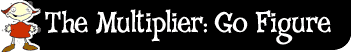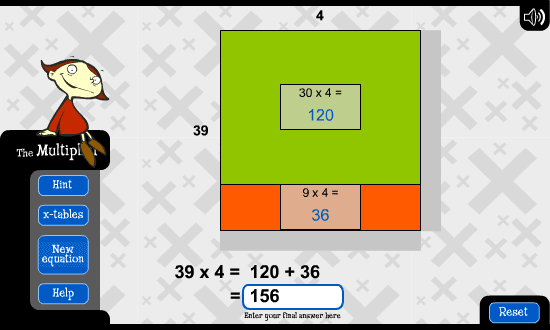﻿ The multiplier: go figure: tens and ones# Tens and ones

The idea behind this strategy is to break up the numbers into other numbers that are easier to multiply.

For example, let's look at 39 times 4.

I could break up the difficult looking number 39 into its tens and ones: 30 and 9. I could then work out 30 times 4, and 9 times 4.

30 times 4 is 120 (because I know 3 times 4 is 12).

9 times 4 is 36.

I would then add 120 and 36 together to get my answer: 156.I could use the tens and ones strategy for a harder equation like 22 times 38. This time I would break both numbers into tens and ones.

The 22 becomes 20 and 2, and the 38 becomes 30 and 8

22 times 38 is broken into 4 easier multiplications:20 times 30;

20 times 8;

2 times 30; and

20 times 30 is?

20 times 8 is?

2 times 30 is?

2 times 8 is?